Examples

Chapter 11 Class 10 Constructions [Deleted]
Serial order wise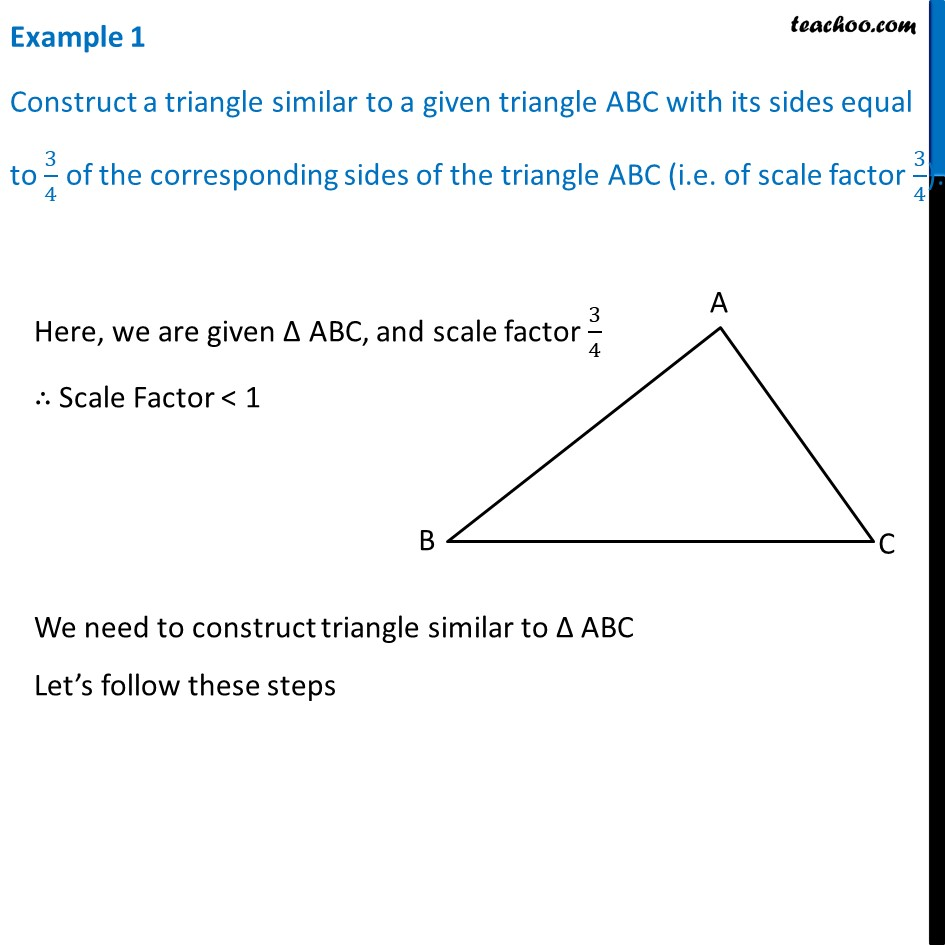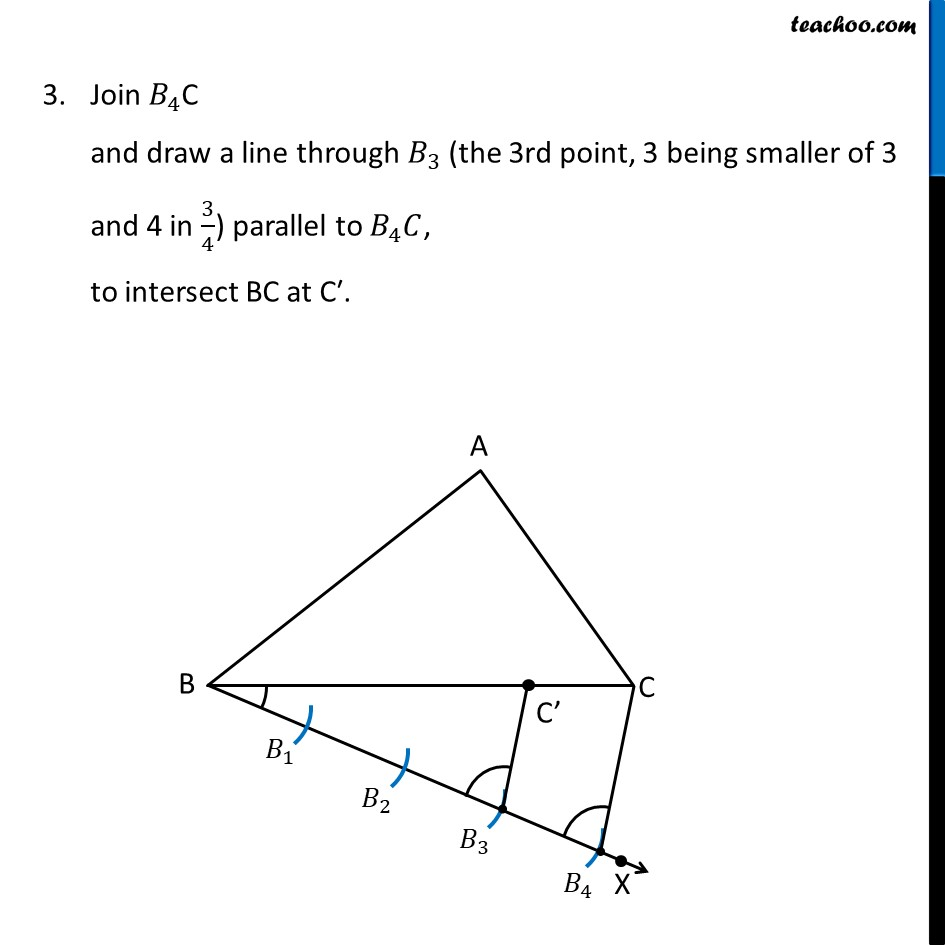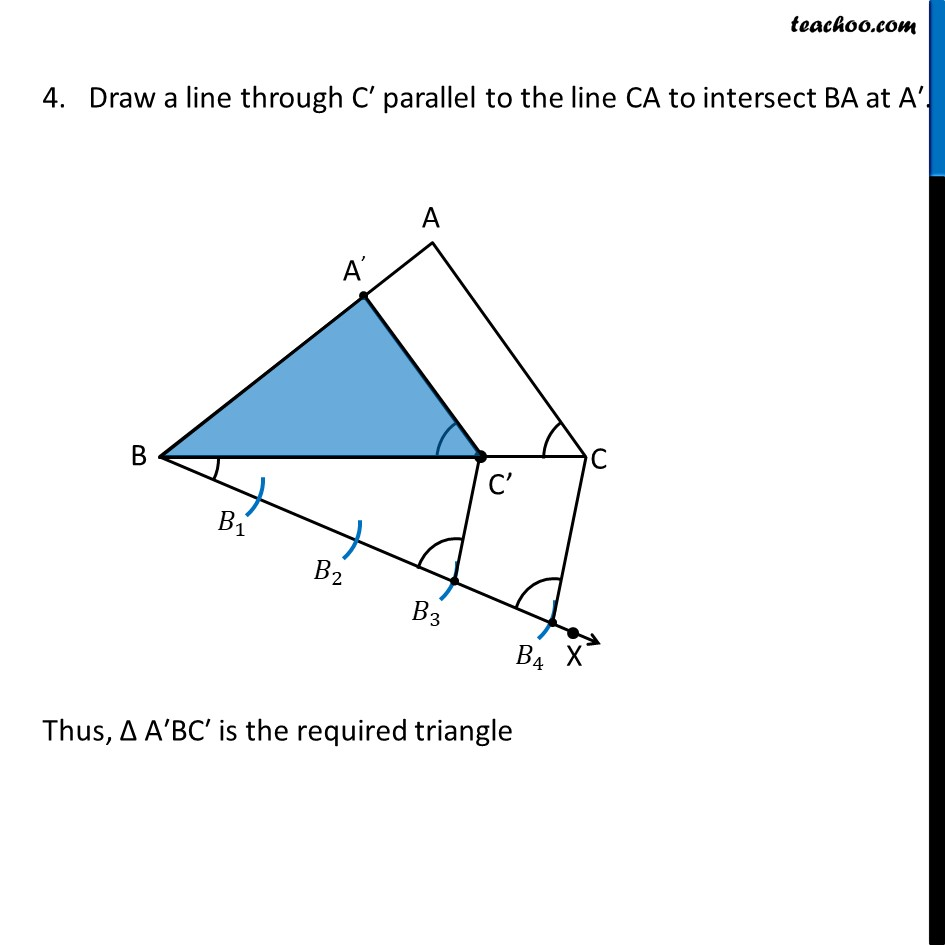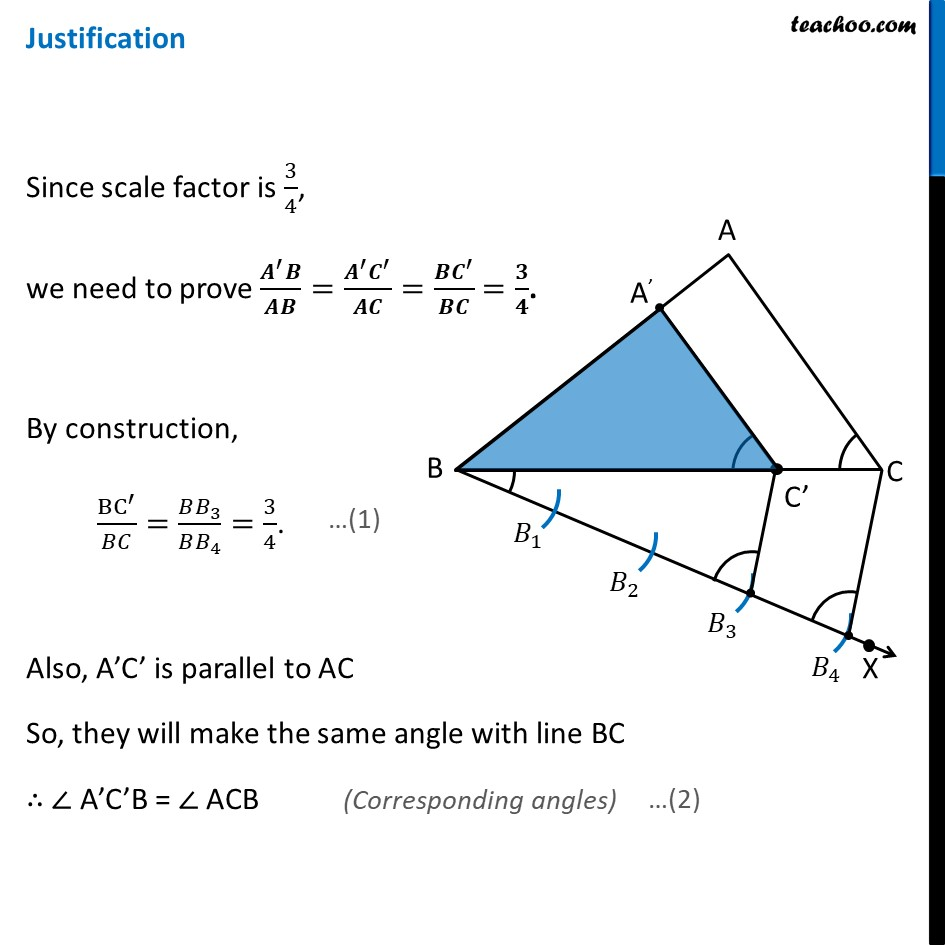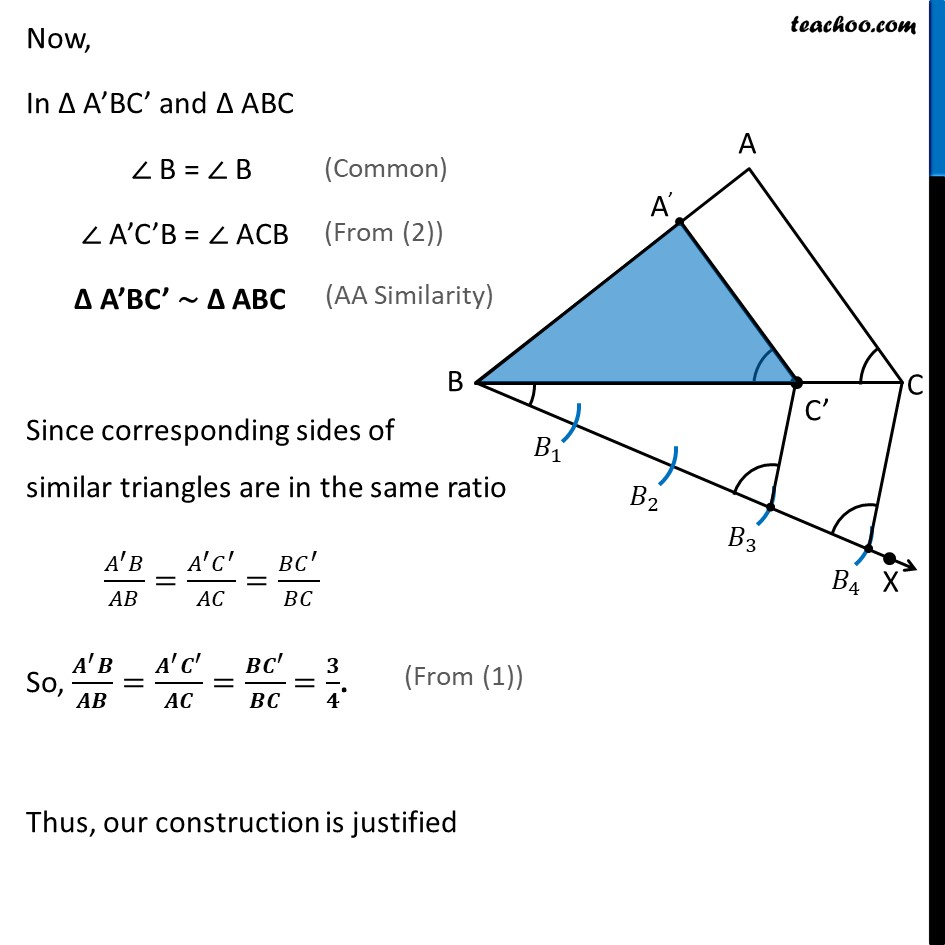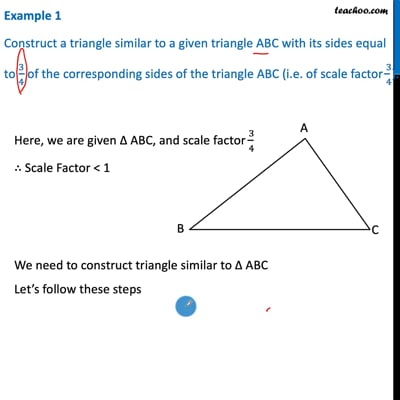This video is only available for Teachoo black users

Maths Crash Course - Live lectures + all videos + Real time Doubt solving!

### Transcript

Example 1 Construct a triangle similar to a given triangle ABC with its sides equal to 3/4 of the corresponding sides of the triangle ABC (i.e. of scale factor 3/4). Here, we are given Δ ABC, and scale factor 3/4 ∴ Scale Factor < 1 We need to construct triangle similar to Δ ABC Let’s follow these steps Steps of construction Draw any ray BX making an acute angle with BC on the side opposite to the vertex A. Mark 4 (the greater of 3 and 4 in 3/4 ) points 𝐵_1,𝐵_2,𝐵_3 and 𝐵_4 on BX so that 〖𝐵𝐵〗_1=𝐵_1 𝐵_2=𝐵_2 𝐵_3=𝐵_3 𝐵_4. Join 𝐵_4C and draw a line through 𝐵_3 (the 3rd point, 3 being smaller of 3 and 4 in 3/4) parallel to 𝐵_4 𝐶, to intersect BC at C′. 4. Draw a line through C′ parallel to the line CA to intersect BA at A′. Thus, Δ A′BC′ is the required triangle Justification Since scale factor is 3/4, we need to prove (𝑨^′ 𝑩)/𝑨𝑩=(𝑨^′ 𝑪^′)/𝑨𝑪=(𝑩𝑪^′)/𝑩𝑪 =𝟑/𝟒. By construction, BC^′/𝐵𝐶=(𝐵𝐵_3)/(𝐵𝐵_4 )=3/4. Also, A’C’ is parallel to AC So, they will make the same angle with line BC ∴ ∠ A’C’B = ∠ ACB Now, In Δ A’BC’ and Δ ABC ∠ B = ∠ B ∠ A’C’B = ∠ ACB Δ A’BC’ ∼ Δ ABC Since corresponding sides of similar triangles are in the same ratio (𝐴^′ 𝐵)/𝐴𝐵=(𝐴^′ 𝐶^′)/𝐴𝐶=(𝐵𝐶^′)/𝐵𝐶 So, (𝑨^′ 𝑩)/𝑨𝑩=(𝑨^′ 𝑪^′)/𝑨𝑪=(𝑩𝑪^′)/𝑩𝑪 =𝟑/𝟒. Thus, our construction is justified